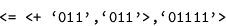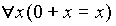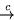Contents

2.3. Automated Theorem Proving

Using formal logic is not the only way to prove theorems in computers, especially those of primary interest for computer scientists. Metacomputation provides an alternative method of automated theorem proving. We face here two different paradigms.

In the axioms-and-logic paradigm of mathematics, we deal with things which are completely undefined, true abstractions. We can know about these things only as much as we can extract from the axioms we have chosen to assume. Mathematical objects - as long as the mathematician is faithful to the proclaimed axioms-and-logic paradigm - do not really exist. The meaning of the statement that certain mathematical objects exist is simply that no logical derivation using these objects leads to contradiction.

In contrast, when we are doing computer science we deal with well-defined finite cybernetic systems, such as Turing machines or computers, and with computational processes in these systems. Compare the treatment of the primary theoretical objects of all exact sciences - natural numbers - in the axiomatic and cybernetic paradigms. In axiomatic arithmetics, numbers are abstract entities operations on which meet requirements codified in a certain number of axioms. In particular, the operation of addition + is defined by two axioms:

 x  +  0  =  x x  +  y' =  (x  +  y )'

where x' is the function 'next number' applied to x. To prove a proposition one must construct, according to well-known rules, a demonstration, which is a sequence of propositions. In the cybernetic paradigm natural numbers are chains of some pieces of matter called symbols, and functions are machines which know how to handle symbols. The Refal program for + is:

 <+ x,'0'> = x <+ x,y'1'> = <+ x,y>'1'

Another function we want to compute is the predicate of equality:

 <= '0','0'> = T <= '0',y'1'> = F <= x'1','0'> = F <= x'1',y'1'> = <= x,y>

The strong side of the axioms-and-logic method is its wide applicability. A theory may be developed about objects (such as those of geometry or set theory) which are not easy to represent by symbolic expressions. Also, the same theorem can be used with different interpretations. Group theory is usually adduced as an example. However, in computer science it is exactly the world of symbolic expressions and processes that we are primarily interested in. For this world the cybernetic paradigm is pretty natural.

The obvious advantage of the cybernetic paradigm is the completely mechanized way to perform computations. To prove that 2+2 = 4, we only have to compute the truth-value of the proposition:When we give this job to the Refal machine, the results is a finite computation process. It ends with T, which proves the statement. This has been a proposition without quantification. Can general propositions be proven by computation? Not directly. But they can be proven by metacomputation. Let us take a simple example from arithmetics where the proof requires the use of mathematical induction. Consider the following statement:(3)

Let us see how this theorem is proven by a supercompiler. The translation of the statement into the cybernetic paradigm is as follows. The initial configuration:

: <= <+ '0',x>, x>

evaluated by the Refal machine with any value substituted for x results in T; so we expect from the supercompiler that it will equivalently transform  into just T.

The supercompiler that makes this job uses the outside-in (lazy) driving. It attempts to drive the call of =, but the nested call of + is a hindrance, thus SCP switches to driving it. Two contractions are produced in accordance with the definition of +: x'0' and xx'1'. With the first contraction the computation is straightforward and leads to T. With the second contraction the machine makes a step in the computation of <+ '0',x'1'>, which produces the configuration:

: <= <+ '0',x>'1', x'1'>

The process returns to the outermost call and makes, in a unique way, one step according to the definition of =. The result is the same as . Thus we have this transition graph:

  x'0'; T + xx'1'; 

The supercompiler easily recognizes such a graph as transformable to just T, since the only exit configuration is T; this is the form which mathematical induction takes in metacomputation. The theorem is proven. (We set aside the problem of termination. In our case it is secured by the fact that all functions involved are total). The associativity of addition is also easily provable in this way. However, proving the commutativity of addition requires more sophisticated techniques, which I shall discuss in Sec. 3.1.

Contents   Next